# Losses¶

All loss functions are used as follows:

``````from pytorch_metric_learning import losses
loss_func = losses.SomeLoss()
loss = loss_func(embeddings, labels) # in your training for-loop
``````

Or if you are using a loss in conjunction with a miner:

``````from pytorch_metric_learning import miners
miner_func = miners.SomeMiner()
loss_func = losses.SomeLoss()
miner_output = miner_func(embeddings, labels) # in your training for-loop
loss = loss_func(embeddings, labels, miner_output)
``````

For some losses, you don't need to pass in labels if you are already passing in pair/triplet indices:

``````loss = loss_func(embeddings, indices_tuple=pairs)
# it also works with ref_emb
loss = loss_func(embeddings, indices_tuple=pairs, ref_emb=ref_emb)
``````

Losses for which you can pass in `indices_tuple` without `labels`
• CircleLoss
• ContrastiveLoss
• IntraPairVarianceLoss
• GeneralizedLiftedStructureLoss
• LiftedStructureLoss
• MarginLoss
• MultiSimilarityLoss
• NTXentLoss
• SignalToNoiseRatioContrastiveLoss
• SupConLoss
• TripletMarginLoss
• TupletMarginLoss

You can specify how losses get reduced to a single value by using a reducer:

``````from pytorch_metric_learning import reducers
reducer = reducers.SomeReducer()
loss_func = losses.SomeLoss(reducer=reducer)
loss = loss_func(embeddings, labels) # in your training for-loop
``````

For tuple losses, you can separate the source of anchors and positives/negatives:

``````loss_func = losses.SomeLoss()
# anchors will come from embeddings
# positives/negatives will come from ref_emb
loss = loss_func(embeddings, labels, ref_emb=ref_emb, ref_labels=ref_labels)
``````

For classification losses, you can get logits using the `get_logits` function:

``````loss_func = losses.SomeClassificationLoss()
logits = loss_func.get_logits(embeddings)
``````

## AngularLoss¶

``````losses.AngularLoss(alpha=40, **kwargs)
``````
Equation: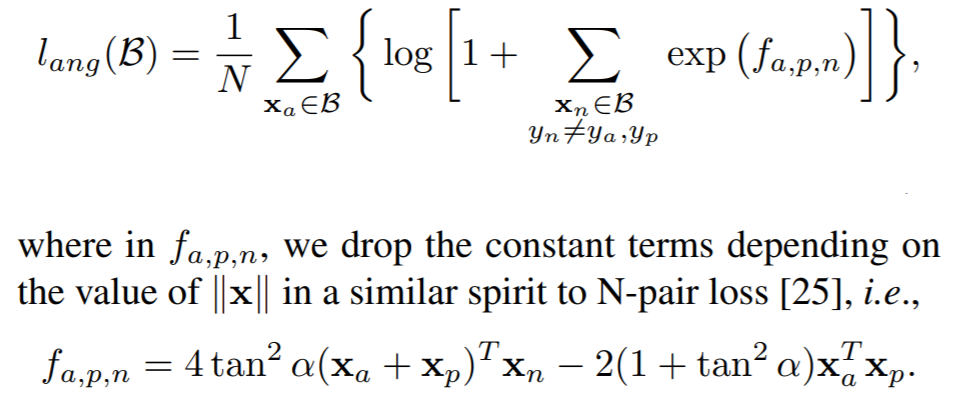Parameters:

• alpha: The angle specified in degrees. The paper uses values between 36 and 55.

Default distance:

Default reducer:

Reducer input:

• loss: The loss for every `a1`, where `(a1,p)` represents every positive pair in the batch. Reduction type is `"element"`.

## ArcFaceLoss¶

ArcFace: Additive Angular Margin Loss for Deep Face Recognition

``````losses.ArcFaceLoss(num_classes, embedding_size, margin=28.6, scale=64, **kwargs)
``````

Equation: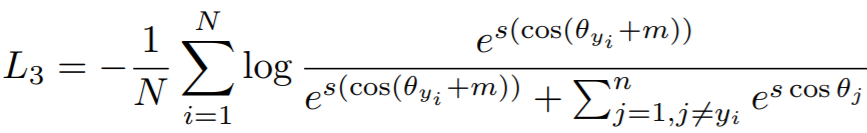Parameters:

• num_classes: The number of classes in your training dataset.
• embedding_size: The size of the embeddings that you pass into the loss function. For example, if your batch size is 128 and your network outputs 512 dimensional embeddings, then set `embedding_size` to 512.
• margin: The angular margin penalty in degrees. In the above equation, `m = radians(margin)`. The paper uses 0.5 radians, which is 28.6 degrees.
• scale: This is `s` in the above equation. The paper uses 64.

Other info:

• This also extends WeightRegularizerMixin, so it accepts `weight_regularizer`, `weight_reg_weight`, and `weight_init_func` as optional arguments.
• This loss requires an optimizer. You need to create an optimizer and pass this loss's parameters to that optimizer. For example:
``````loss_func = losses.ArcFaceLoss(...).to(torch.device('cuda'))
loss_optimizer = torch.optim.SGD(loss_func.parameters(), lr=0.01)
# then during training:
loss_optimizer.step()
``````

Default distance:

Default reducer:

Reducer input:

• loss: The loss per element in the batch. Reduction type is `"element"`.

## BaseMetricLossFunction¶

All loss functions extend this class and therefore inherit its `__init__` parameters.

``````losses.BaseMetricLossFunction(collect_stats = False,
reducer = None,
distance = None,
embedding_regularizer = None,
embedding_reg_weight = 1)
``````

Parameters:

• collect_stats: If True, will collect various statistics that may be useful to analyze during experiments. If False, these computations will be skipped. Want to make `True` the default? Set the global COLLECT_STATS flag.
• reducer: A reducer object. If None, then the default reducer will be used.
• distance: A distance object. If None, then the default distance will be used.
• embedding_regularizer: A regularizer object that will be applied to embeddings. If None, then no embedding regularization will be used.
• embedding_reg_weight: If an embedding regularizer is used, then its loss will be multiplied by this amount before being added to the total loss.

Default distance:

Default reducer:

Reducer input:

• embedding_reg_loss: Only exists if an embedding regularizer is used. It contains the loss per element in the batch. Reduction type is `"already_reduced"`.

Required Implementations:

``````def compute_loss(self, embeddings, labels, indices_tuple, ref_emb, ref_labels):
raise NotImplementedError
``````

## CentroidTripletLoss¶

On the Unreasonable Effectiveness of Centroids in Image Retrieval

This is like TripletMarginLoss, except the positives and negatives are class centroids.

``````losses.CentroidTripletLoss(margin=0.05,
swap=False,
smooth_loss=False,
triplets_per_anchor="all",
**kwargs)
``````

Unlike many other losses, the instance of this class can only be called as the following:

``````from pytorch_metric_learning import losses
loss_func = losses.CentroidTripletLoss()
loss = loss_func(embeddings, labels)
``````

and does not allow for use of `ref_embs`, `ref_labels`. Furthermore, there must be at least 2 embeddings associated with each label. Refer to this issue for details.

Parameters:

Default distance:

Default reducer:

## CircleLoss¶

Circle Loss: A Unified Perspective of Pair Similarity Optimization

``````losses.CircleLoss(m=0.4, gamma=80, **kwargs)
``````

Equations: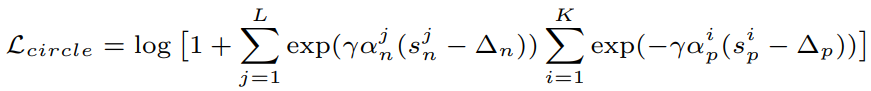where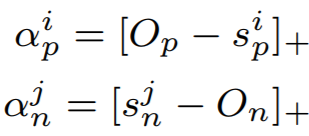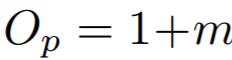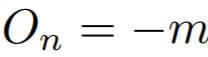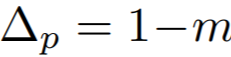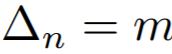Parameters:

• m: The relaxation factor that controls the radius of the decision boundary. The paper uses 0.25 for face recognition, and 0.4 for fine-grained image retrieval (images of birds, cars, and online products).
• gamma: The scale factor that determines the largest scale of each similarity score. The paper uses 256 for face recognition, and 80 for fine-grained image retrieval.

Default distance:

Default reducer:

Reducer input:

• loss: The loss per element in the batch. Reduction type is `"element"`.

## ContrastiveLoss¶

``````losses.ContrastiveLoss(pos_margin=0, neg_margin=1, **kwargs):
``````

Equation:

If using a distance metric like LpDistance, the loss is: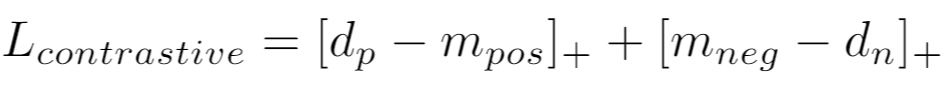If using a similarity metric like CosineSimilarity, the loss is: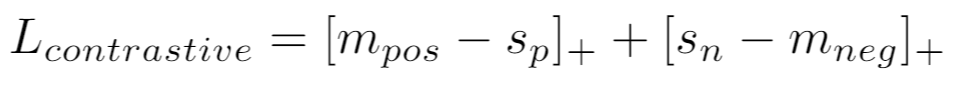Parameters:

• pos_margin: The distance (or similarity) over (under) which positive pairs will contribute to the loss.
• neg_margin: The distance (or similarity) under (over) which negative pairs will contribute to the loss.

Note that the default values for `pos_margin` and `neg_margin` are suitable if you are using a non-inverted distance measure, like LpDistance. If you use an inverted distance measure like CosineSimilarity, then more appropriate values would be `pos_margin = 1` and `neg_margin = 0`.

Default distance:

Default reducer:

Reducer input:

• pos_loss: The loss per positive pair in the batch. Reduction type is `"pos_pair"`.
• neg_loss: The loss per negative pair in the batch. Reduction type is `"neg_pair"`.

## CosFaceLoss¶

CosFace: Large Margin Cosine Loss for Deep Face Recognition

``````losses.CosFaceLoss(num_classes, embedding_size, margin=0.35, scale=64, **kwargs)
``````

Equation: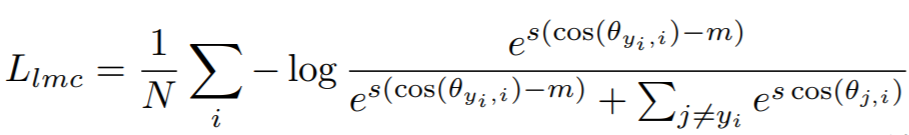Parameters:

• num_classes: The number of classes in your training dataset.
• embedding_size: The size of the embeddings that you pass into the loss function. For example, if your batch size is 128 and your network outputs 512 dimensional embeddings, then set `embedding_size` to 512.
• margin: The cosine margin penalty (m in the above equation). The paper used values between 0.25 and 0.45.
• scale: This is `s` in the above equation. The paper uses 64.

Other info:

• This also extends WeightRegularizerMixin, so it accepts `weight_regularizer`, `weight_reg_weight`, and `weight_init_func` as optional arguments.
• This loss requires an optimizer. You need to create an optimizer and pass this loss's parameters to that optimizer. For example:
``````loss_func = losses.CosFaceLoss(...).to(torch.device('cuda'))
loss_optimizer = torch.optim.SGD(loss_func.parameters(), lr=0.01)
# then during training:
loss_optimizer.step()
``````

Default distance:

Default reducer:

Reducer input:

• loss: The loss per element in the batch. Reduction type is `"element"`.

## CrossBatchMemory¶

This wraps a loss function, and implements Cross-Batch Memory for Embedding Learning. It stores embeddings from previous iterations in a queue, and uses them to form more pairs/triplets with the current iteration's embeddings.

``````losses.CrossBatchMemory(loss, embedding_size, memory_size=1024, miner=None)
``````

Parameters:

• loss: The loss function to be wrapped. For example, you could pass in `ContrastiveLoss()`.
• embedding_size: The size of the embeddings that you pass into the loss function. For example, if your batch size is 128 and your network outputs 512 dimensional embeddings, then set `embedding_size` to 512.
• memory_size: The size of the memory queue.
• miner: An optional tuple miner, which will be used to mine pairs/triplets from the memory queue.

Forward function

``````loss_fn(embeddings, labels, indices_tuple=None, enqueue_idx=None)
``````

As shown above, CrossBatchMemory comes with a 4th argument in its `forward` function:

• enqueue_idx: The indices of `embeddings` that will be added to the memory queue. In other words, only `embeddings[enqueue_idx]` will be added to memory. This enables CrossBatchMemory to be used in self-supervision frameworks like MoCo. Check out the MoCo on CIFAR100 notebook to see how this works.

Reset queue

The queue can be cleared like this:

``````loss_fn.reset_queue()
``````

## FastAPLoss¶

Deep Metric Learning to Rank

``````losses.FastAPLoss(num_bins=10, **kwargs)
``````

Parameters:

• num_bins: The number of soft histogram bins for calculating average precision. The paper suggests using 10.

Default distance:

Default reducer:

Reducer input:

• loss: The loss per element that has at least 1 positive in the batch. Reduction type is `"element"`.

## GenericPairLoss¶

``````losses.GenericPairLoss(mat_based_loss, **kwargs)
``````
Parameters:

• mat_based_loss: See required implementations.

Required Implementations:

``````# If mat_based_loss is True, then this takes in mat, pos_mask, neg_mask
# If False, this takes in pos_pair, neg_pair, indices_tuple
def _compute_loss(self):
raise NotImplementedError
``````

## GeneralizedLiftedStructureLoss¶

This was presented in In Defense of the Triplet Loss for Person Re-Identification. It is a modification of the original LiftedStructureLoss

``````losses.GeneralizedLiftedStructureLoss(neg_margin=1, pos_margin=0, **kwargs)
``````
Equation: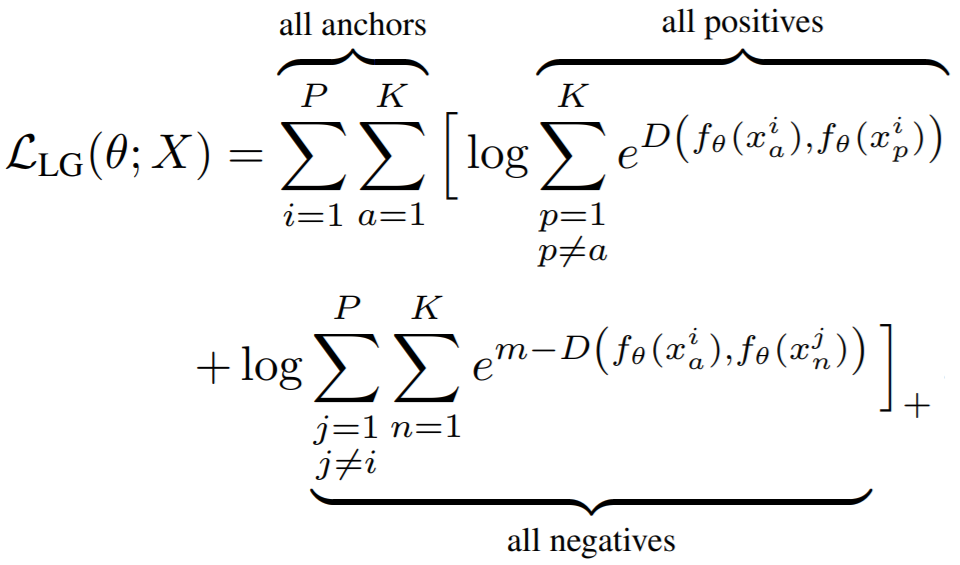Parameters:

• pos_margin: The margin in the expression `e^(D - margin)`. The paper uses `pos_margin = 0`, which is why this margin does not appear in the above equation.
• neg_margin: This is `m` in the above equation. The paper used values between 0.1 and 1.

Default distance:

Default reducer:

Reducer input:

• loss: The loss per element in the batch. Reduction type is `"element"`.

## InstanceLoss¶

``````losses.InstanceLoss(gamma=64, **kwargs)
``````

Parameters:

• gamma: The cosine similarity matrix is scaled by this amount.

## IntraPairVarianceLoss¶

``````losses.IntraPairVarianceLoss(pos_eps=0.01, neg_eps=0.01, **kwargs)
``````

Equations: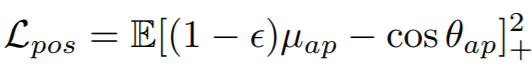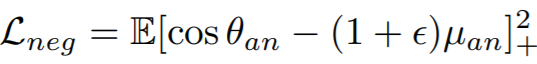Parameters:

• pos_eps: The epsilon in the Lpos equation. The paper uses 0.01.
• neg_eps: The epsilon in the Lneg equation. The paper uses 0.01.

You should probably use this in conjunction with another loss, as described in the paper. You can accomplish this by using MultipleLosses:

``````main_loss = losses.TupletMarginLoss()
var_loss = losses.IntraPairVarianceLoss()
complete_loss = losses.MultipleLosses([main_loss, var_loss], weights=[1, 0.5])
``````

Default distance:

Default reducer:

Reducer input:

• pos_loss: The loss per positive pair in the batch. Reduction type is `"pos_pair"`.
• neg_loss: The loss per negative pair in the batch. Reduction type is `"neg_pair"`.

## LargeMarginSoftmaxLoss¶

Large-Margin Softmax Loss for Convolutional Neural Networks

``````losses.LargeMarginSoftmaxLoss(num_classes,
embedding_size,
margin=4,
scale=1,
**kwargs)
``````

Equations: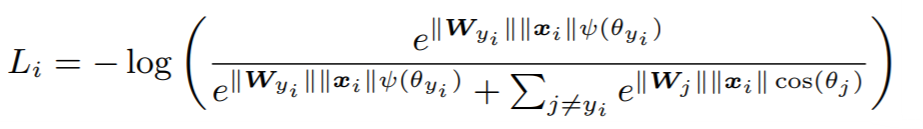where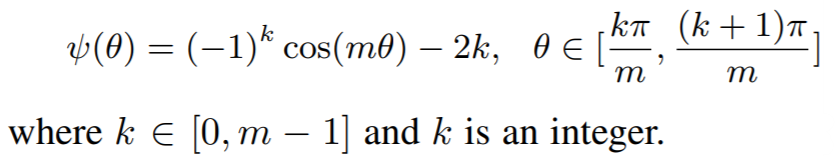Parameters:

• num_classes: The number of classes in your training dataset.
• embedding_size: The size of the embeddings that you pass into the loss function. For example, if your batch size is 128 and your network outputs 512 dimensional embeddings, then set `embedding_size` to 512.
• margin: An integer which dictates the size of the angular margin. This is `m` in the above equation. The paper finds `m=4` works best.
• scale: The exponent multiplier in the loss's softmax expression. The paper uses `scale = 1`, which is why it does not appear in the above equation.

Other info:

• This also extends WeightRegularizerMixin, so it accepts `weight_regularizer`, `weight_reg_weight`, and `weight_init_func` as optional arguments.
• This loss requires an optimizer. You need to create an optimizer and pass this loss's parameters to that optimizer. For example:
``````loss_func = losses.LargeMarginSoftmaxLoss(...).to(torch.device('cuda'))
loss_optimizer = torch.optim.SGD(loss_func.parameters(), lr=0.01)
# then during training:
loss_optimizer.step()
``````

Default distance:

Default reducer:

Reducer input:

• loss: The loss per element in the batch. Reduction type is `"element"`.

## LiftedStructureLoss¶

The original lifted structure loss as presented in Deep Metric Learning via Lifted Structured Feature Embedding

``````losses.LiftedStructureLoss(neg_margin=1, pos_margin=0, **kwargs):
``````

Equation: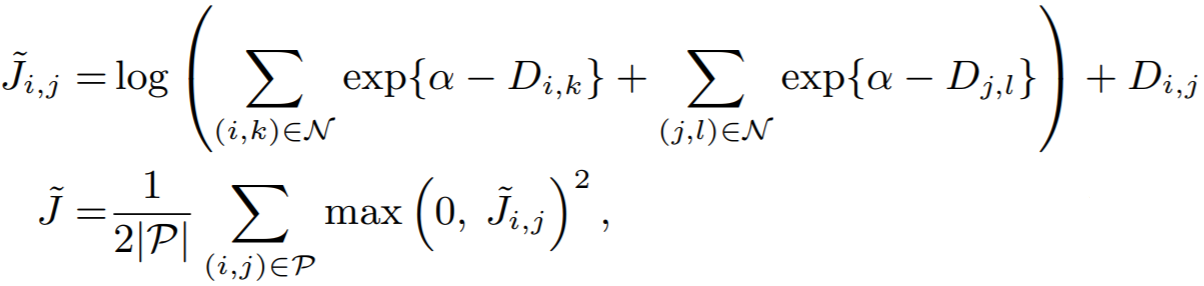Parameters:

• pos_margin: The margin in the expression `D_(i,j) - margin`. The paper uses `pos_margin = 0`, which is why it does not appear in the above equation.
• neg_margin: This is `alpha` in the above equation. The paper uses 1.

Default distance:

Default reducer:

Reducer input:

• loss: The loss per positive pair in the batch. Reduction type is `"pos_pair"`.

## MarginLoss¶

``````losses.MarginLoss(margin=0.2,
nu=0,
beta=1.2,
triplets_per_anchor="all",
learn_beta=False,
num_classes=None,
**kwargs)
``````

Equations: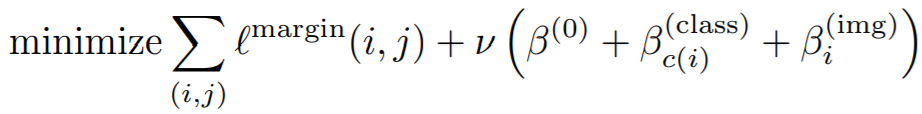where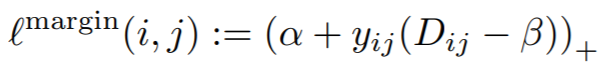Parameters:

• margin: This is alpha in the above equation. The paper uses 0.2.
• nu: The regularization weight for the magnitude of beta.
• beta: This is beta in the above equation. The paper uses 1.2 as the initial value.
• triplets_per_anchor: The number of triplets per element to sample within a batch. Can be an integer or the string "all". For example, if your batch size is 128, and triplets_per_anchor is 100, then 12800 triplets will be sampled. If triplets_per_anchor is "all", then all possible triplets in the batch will be used.
• learn_beta: If True, beta will be a torch.nn.Parameter, which can be optimized using any PyTorch optimizer.
• num_classes: If not None, then beta will be of size `num_classes`, so that a separate beta is used for each class during training.

Default distance:

Default reducer:

Reducer input:

• margin_loss: The loss per triplet in the batch. Reduction type is `"triplet"`.
• beta_reg_loss: The regularization loss per element in `self.beta`. Reduction type is `"already_reduced"` if `self.num_classes = None`. Otherwise it is `"element"`.

## MultipleLosses¶

This is a simple wrapper for multiple losses. Pass in a list of already-initialized loss functions. Then, when you call forward on this object, it will return the sum of all wrapped losses.

``````losses.MultipleLosses(losses, miners=None, weights=None)
``````
Parameters:

• losses: A list or dictionary of initialized loss functions. On the forward call of MultipleLosses, each wrapped loss will be computed, and then the average will be returned.
• miners: Optional. A list or dictionary of mining functions. This allows you to pair mining functions with loss functions. For example, if `losses = [loss_A, loss_B]`, and `miners = [None, miner_B]` then no mining will be done for `loss_A`, but the output of `miner_B` will be passed to `loss_B`. The same logic applies if `losses = {"loss_A": loss_A, "loss_B": loss_B}` and `miners = {"loss_B": miner_B}`.
• weights: Optional. A list or dictionary of loss weights, which will be multiplied by the corresponding losses obtained by the loss functions. The default is to multiply each loss by 1. If `losses` is a list, then `weights` must be a list. If `losses` is a dictionary, `weights` must contain the same keys as `losses`.

## MultiSimilarityLoss¶

``````losses.MultiSimilarityLoss(alpha=2, beta=50, base=0.5, **kwargs)
``````

Equation: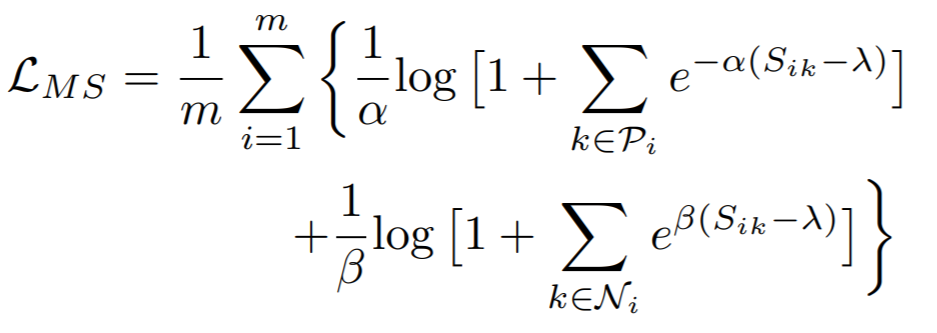Parameters:

• alpha: The weight applied to positive pairs. The paper uses 2.
• beta: The weight applied to negative pairs. The paper uses 50.
• base: The offset applied to the exponent in the loss. This is lambda in the above equation. The paper uses 1.

Default distance:

Default reducer:

Reducer input:

• loss: The loss per element in the batch. Reduction type is `"element"`.

## NCALoss¶

``````losses.NCALoss(softmax_scale=1, **kwargs)
``````

Equations: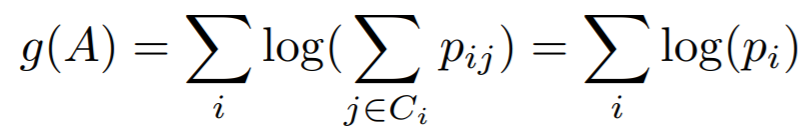where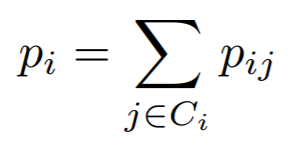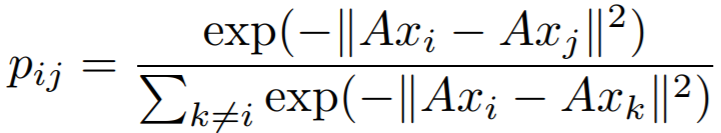In this implementation, we use `-g(A)` as the loss.

Parameters:

• softmax_scale: The exponent multiplier in the loss's softmax expression. The paper uses `softmax_scale = 1`, which is why it does not appear in the above equations.

Default distance:

Default reducer:

Reducer input:

• loss: The loss per element in the batch, that results in a non zero exponent in the cross entropy expression. Reduction type is `"element"`.

## NormalizedSoftmaxLoss¶

``````losses.NormalizedSoftmaxLoss(num_classes, embedding_size, temperature=0.05, **kwargs)
``````

Equation: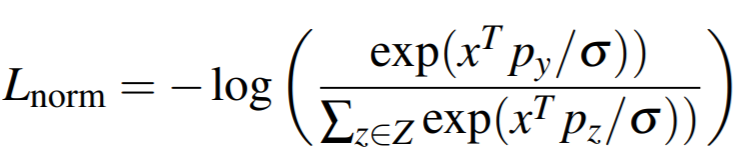Parameters:

• num_classes: The number of classes in your training dataset.
• embedding_size: The size of the embeddings that you pass into the loss function. For example, if your batch size is 128 and your network outputs 512 dimensional embeddings, then set `embedding_size` to 512.
• temperature: This is sigma in the above equation. The paper uses 0.05.

Other info

• This also extends WeightRegularizerMixin, so it accepts `weight_regularizer`, `weight_reg_weight`, and `weight_init_func` as optional arguments.
• This loss requires an optimizer. You need to create an optimizer and pass this loss's parameters to that optimizer. For example:
``````loss_func = losses.NormalizedSoftmaxLoss(...).to(torch.device('cuda'))
loss_optimizer = torch.optim.SGD(loss_func.parameters(), lr=0.01)
# then during training:
loss_optimizer.step()
``````

Default distance:

Default reducer:

Reducer input:

• loss: The loss per element in the batch. Reduction type is `"element"`.

## NPairsLoss¶

Improved Deep Metric Learning with Multi-class N-pair Loss Objective

If your batch has more than 2 samples per label, then you should use NTXentLoss.

``````losses.NPairsLoss(**kwargs)
``````

Default distance:

Default reducer:

Reducer input:

• loss: The loss per element in the batch. Reduction type is `"element"`.

## NTXentLoss¶

This is also known as InfoNCE, and is a generalization of the NPairsLoss. It has been used in self-supervision papers such as:

Equation: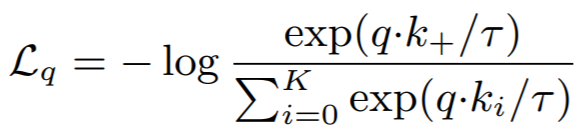Parameters:

• temperature: This is tau in the above equation. The MoCo paper uses 0.07, while SimCLR uses 0.5.

Default distance:

Default reducer:

Reducer input:

• loss: The loss per positive pair in the batch. Reduction type is `"pos_pair"`.

## ProxyAnchorLoss¶

``````losses.ProxyAnchorLoss(num_classes, embedding_size, margin = 0.1, alpha = 32, **kwargs)
``````

Equation: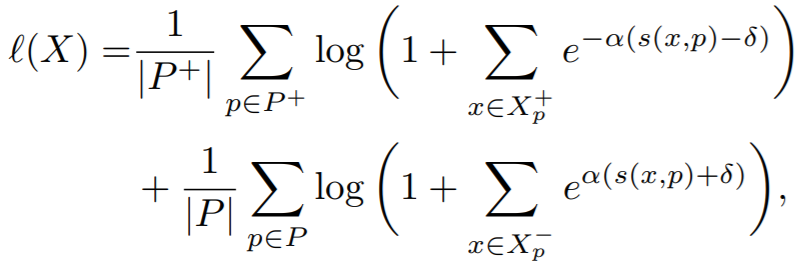Parameters:

• num_classes: The number of classes in your training dataset.
• embedding_size: The size of the embeddings that you pass into the loss function. For example, if your batch size is 128 and your network outputs 512 dimensional embeddings, then set `embedding_size` to 512.
• margin: This is delta in the above equation. The paper uses 0.1.
• alpha: This is alpha in the above equation. The paper uses 32.

Other info

• This also extends WeightRegularizerMixin, so it accepts `weight_regularizer`, `weight_reg_weight`, and `weight_init_func` as optional arguments.
• This loss requires an optimizer. You need to create an optimizer and pass this loss's parameters to that optimizer. For example:
``````loss_func = losses.ProxyAnchorLoss(...).to(torch.device('cuda'))
loss_optimizer = torch.optim.SGD(loss_func.parameters(), lr=0.01)
# then during training:
loss_optimizer.step()
``````

Default distance:

Default reducer:

Reducer input:

• pos_loss: The positive pair loss per proxy. Reduction type is `"element"`.
• neg_loss: The negative pair loss per proxy. Reduction type is `"element"`.

## ProxyNCALoss¶

``````losses.ProxyNCALoss(num_classes, embedding_size, softmax_scale=1, **kwargs)
``````

Parameters:

• num_classes: The number of classes in your training dataset.
• embedding_size: The size of the embeddings that you pass into the loss function. For example, if your batch size is 128 and your network outputs 512 dimensional embeddings, then set `embedding_size` to 512.
• softmax_scale: See NCALoss

Other info

• This also extends WeightRegularizerMixin, so it accepts `weight_regularizer`, `weight_reg_weight`, and `weight_init_func` as optional arguments.
• This loss requires an optimizer. You need to create an optimizer and pass this loss's parameters to that optimizer. For example:
``````loss_func = losses.ProxyNCALoss(...).to(torch.device('cuda'))
loss_optimizer = torch.optim.SGD(loss_func.parameters(), lr=0.01)
# then during training:
loss_optimizer.step()
``````

Default distance:

Default reducer:

Reducer input:

• loss: The loss per element in the batch, that results in a non zero exponent in the cross entropy expression. Reduction type is `"element"`.

## SignalToNoiseRatioContrastiveLoss¶

``````losses.SignalToNoiseRatioContrastiveLoss(pos_margin=0, neg_margin=1, **kwargs):
``````

Parameters:

• pos_margin: The noise-to-signal ratio over which positive pairs will contribute to the loss.
• neg_margin: The noise-to-signal ratio under which negative pairs will contribute to the loss.

Default distance:

Default reducer:

Reducer input:

• pos_loss: The loss per positive pair in the batch. Reduction type is `"pos_pair"`.
• neg_loss: The loss per negative pair in the batch. Reduction type is `"neg_pair"`.

## SoftTripleLoss¶

``````losses.SoftTripleLoss(num_classes,
embedding_size,
centers_per_class=10,
la=20,
gamma=0.1,
margin=0.01,
**kwargs)
``````

Equations: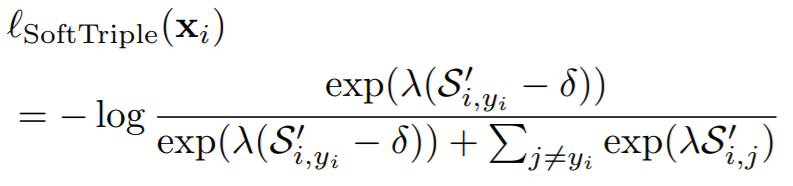where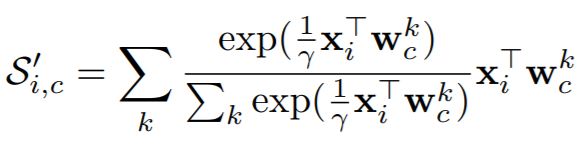Parameters:

• num_classes: The number of classes in your training dataset.
• embedding_size: The size of the embeddings that you pass into the loss function. For example, if your batch size is 128 and your network outputs 512 dimensional embeddings, then set `embedding_size` to 512.
• centers_per_class: The number of weight vectors per class. (The regular cross entropy loss has 1 center per class.) The paper uses 10.
• la: This is lambda in the above equation.
• gamma: This is gamma in the above equation. The paper uses 0.1.
• margin: The is delta in the above equations. The paper uses 0.01.

Other info

• This also extends WeightRegularizerMixin, so it accepts `weight_regularizer`, `weight_reg_weight`, and `weight_init_func` as optional arguments.
• This loss requires an optimizer. You need to create an optimizer and pass this loss's parameters to that optimizer. For example:
``````loss_func = losses.SoftTripleLoss(...).to(torch.device('cuda'))
loss_optimizer = torch.optim.SGD(loss_func.parameters(), lr=0.01)
# then during training:
loss_optimizer.step()
``````

Default distance:

Default reducer:

Reducer input:

• loss: The loss per element in the batch. Reduction type is `"element"`.

## SphereFaceLoss¶

SphereFace: Deep Hypersphere Embedding for Face Recognition

``````losses.SphereFaceLoss(num_classes,
embedding_size,
margin=4,
scale=1,
**kwargs)
``````

Parameters:

Other info

• This also extends WeightRegularizerMixin, so it accepts `weight_regularizer`, `weight_reg_weight`, and `weight_init_func` as optional arguments.
• This loss requires an optimizer. You need to create an optimizer and pass this loss's parameters to that optimizer. For example:
``````loss_func = losses.SphereFaceLoss(...).to(torch.device('cuda'))
loss_optimizer = torch.optim.SGD(loss_func.parameters(), lr=0.01)
# then during training:
loss_optimizer.step()
``````

Default distance:

Default reducer:

Reducer input:

• loss: The loss per element in the batch. Reduction type is `"element"`.

## SubCenterArcFaceLoss¶

Sub-center ArcFace: Boosting Face Recognition by Large-scale Noisy Web Faces

This loss extends ArcFaceLoss. It uses multiple sub centers per class, instead of just a single center, hence the name Sub-center ArcFace.

``````losses.SubCenterArcFaceLoss(
num_classes,
embedding_size,
margin=28.6,
scale=64,
sub_centers=3,
**kwargs
)
``````

Parameters:

• sub_centers: The number of sub centers per class.

See ArcFaceLoss for a description of the other parameters.

Other info:

• This loss requires an optimizer. See ArcFaceLoss for details.
• See ArcFaceLoss for default distance, reducer, and reducer input.

Getting outliers and dominant centers

Outliers and dominant centers can be computed as described in the paper.

``````outliers, dominant_centers = loss_func.get_outliers(
embeddings, labels, threshold=75, return_dominant_centers=True
)
``````

## SupConLoss¶

Described in Supervised Contrastive Learning.

``````losses.SupConLoss(temperature=0.1, **kwargs)
``````

Equation: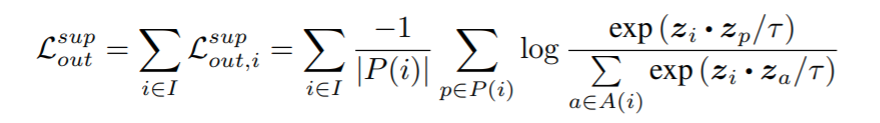Parameters:

• temperature: This is tau in the above equation. The paper uses 0.1.

Default distance:

Default reducer:

Reducer input:

• loss: The loss per element in the batch. If an element has only negative pairs or no pairs, it's ignored thanks to `AvgNonZeroReducer`. Reduction type is `"element"`.

## TripletMarginLoss¶

``````losses.TripletMarginLoss(margin=0.05,
swap=False,
smooth_loss=False,
triplets_per_anchor="all",
**kwargs)
``````

Equation: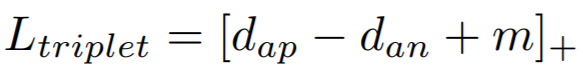Parameters:

• margin: The desired difference between the anchor-positive distance and the anchor-negative distance. This is `m` in the above equation.
• swap: Use the positive-negative distance instead of anchor-negative distance, if it violates the margin more.
• smooth_loss: Use the log-exp version of the triplet loss
• triplets_per_anchor: The number of triplets per element to sample within a batch. Can be an integer or the string "all". For example, if your batch size is 128, and triplets_per_anchor is 100, then 12800 triplets will be sampled. If triplets_per_anchor is "all", then all possible triplets in the batch will be used.

Default distance:

Default reducer:

Reducer input:

• loss: The loss per triplet in the batch. Reduction type is `"triplet"`.

## TupletMarginLoss¶

``````losses.TupletMarginLoss(margin=5.73, scale=64, **kwargs)
``````

Equation: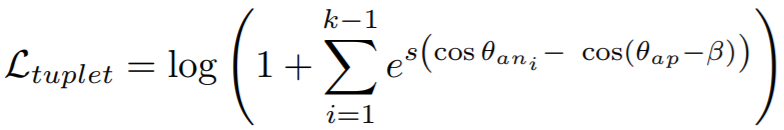Parameters:

• margin: The angular margin (in degrees) applied to positive pairs. This is beta in the above equation. The paper uses a value of 5.73 degrees (0.1 radians).
• scale: This is `s` in the above equation.

The paper combines this loss with IntraPairVarianceLoss. You can accomplish this by using MultipleLosses:

``````main_loss = losses.TupletMarginLoss()
var_loss = losses.IntraPairVarianceLoss()
complete_loss = losses.MultipleLosses([main_loss, var_loss], weights=[1, 0.5])
``````

Default distance:

Default reducer:

Reducer input:

• loss: The loss per positive pair in the batch. Reduction type is `"pos_pair"`.

## WeightRegularizerMixin¶

Losses can extend this class in addition to BaseMetricLossFunction. You should extend this class if your loss function contains a learnable weight matrix.

``````losses.WeightRegularizerMixin(weight_init_func=None, weight_regularizer=None, weight_reg_weight=1, **kwargs)
``````

Parameters:

• weight_init_func: An TorchInitWrapper object, which will be used to initialize the weights of the loss function.
• weight_regularizer: The regularizer to apply to the loss's learned weights.
• weight_reg_weight: The amount the regularization loss will be multiplied by.

Extended by:

## VICRegLoss¶

``````losses.VICRegLoss(invariance_lambda=25,
variance_mu=25,
covariance_v=1,
eps=1e-4,
**kwargs)
``````

Usage:

Unlike other loss functions, `VICRegLoss` does not accept `labels` or `indices_tuple`:

``````loss_fn = VICRegLoss()
loss = loss_fn(embeddings, ref_emb)
``````

Equations: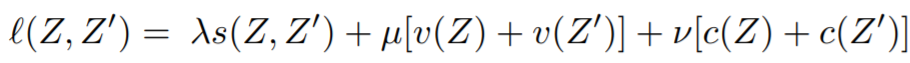where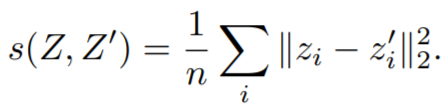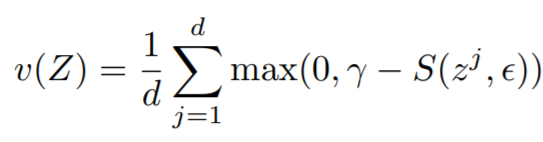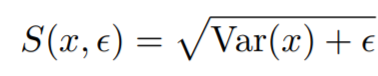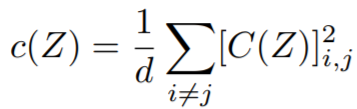Parameters:

• invariance_lambda: The weight of the invariance term.
• variance_mu: The weight of the variance term.
• covariance_v: The weight of the covariance term.
• eps: Small scalar to prevent numerical instability.

Default distance:

• Not applicable. You cannot pass in a distance function.

Default reducer:

Reducer input:

• invariance_loss: The MSE loss between `embeddings[i]` and `ref_emb[i]`. Reduction type is `"element"`.
• variance_loss1: The variance loss for `embeddings`. Reduction type is `"element"`.
• variance_loss2: The variance loss for `ref_emb`. Reduction type is `"element"`.
• covariance_loss: The covariance loss. This loss is already reduced to a single value.